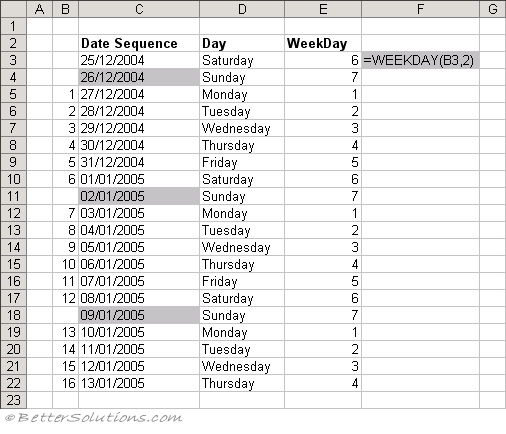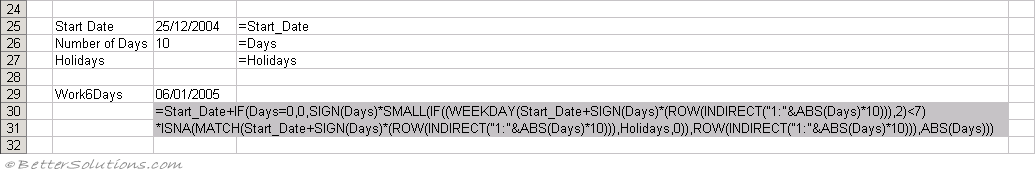# WORKDAY6

Returns the serial number before or after a given number of days from a start date assuming a 6-day working week.
For instructions on how to add a function to a workbook refer to the page under Inserting Functions

`'lStartDate - The starting date.'lDays - The number of non weekend and non holiday days before ot after "lStartDate".'rgeHolidays - The range of cells containing your holidays.Public Function WORKDAY6(ByVal lStartDate As Long, _                          ByVal lDays As Long, _                          ByVal rgeHolidays As Range) As Long Dim ldate As Long Dim idaycount As Long Dim bholiday As Boolean Dim objcell As Range    If lDays > 0 Then idaycount = idaycount + 1    If lDays < 0 Then idaycount = idaycount - 1    Do While (lDays <> 0)       ldate = lStartDate + idaycount       bholiday = False       If WorksheetFunction.Weekday(ldate, 2) < 7 Then          For Each objcell In rgeHolidays             If ldate = CLng(objcell.Value) Then                bholiday = True             End If          Next objcell          If bholiday = False Then             If lDays > 0 Then lDays = lDays - 1             If lDays < 0 Then lDays = lDays + 1          End If       End If       If lDays > 0 Then idaycount = idaycount + 1       If lDays < 0 Then idaycount = idaycount - 1    Loop    WORKDAY6 = lStartDate + idaycount End Function `

This user defined function is similar to the built-in WORKDAY function.
There is an Array Formula equivalent if you do not want to use the user defined function.

### Array Formula

This array formula was originally created by the late Frank Kabel who was an Excel MVP (Microsoft Valued Professional).
To find out more about Array Formulas please refer to the Array Formulas section.
This example illustrates how to use an array formula to obtain the required date.
The table below shows the sequence of dates we are going to use and is just for reference.The formula in cell "C3" returns the next working day (assuming a 6 day working week) that is 10 days after 25/12/2004.
This formula must be entered as an array formula using (Ctrl + Shift + Enter).The named range "Start_Date" refers to cell "C25" which contains your start date.
The named range "Days" refers to the cell "C26" which contains the number of workdays past the start date which you want to return.
The named range "Holidays" refers to the cell "C27" which should contain the dates of any additional dates you want to exclude.
The formula [SIGN(Days)] is used to determine if you want to return a date before or after the start date (i.e. whether to add or subtract the number of days).
The formula [ROW(INDIRECT("1:"&ABS(Days) ) )] returns an array of numbers from 1 to the number of days before or after.
This array is then multiplied by 10. This is to allow for all the weekends and possible holidays that might have to be excluded from the series.

The formula [WEEKDAY(Start_Date+SIGN(Days)*ROW(INDIRECT("1:"&ABS(Days)*10))),2)] returns an array of 1's and 0's indicating if the date is a Sunday.
Using a return-type of "2" for the WEEKDAY function any Sundays will be identified by the number 7.
The formula [MATCH(Start_Date+SIGN(Days)*ROW(INDIRECT("1:"&ABS(Days)*10))),Holidays,0))] creates a similar array of dates and then does a lookup to check if any of these days match the dates in the defined Holidays cell range.
The MATCH function will return the position of any dates which are defined as holidays.
This is then wrapped by a ISNA function because a "#N/A" is returned when there is no match.
The two arrays are then multiplied together to get a single array that contains just 1's and 0's.
The SMALL function is then used to return the date which is in the correct position from this final array.
This formula is extremely complicated so you may prefer to use a custom worksheet function instead.

© 2022 Better Solutions Limited